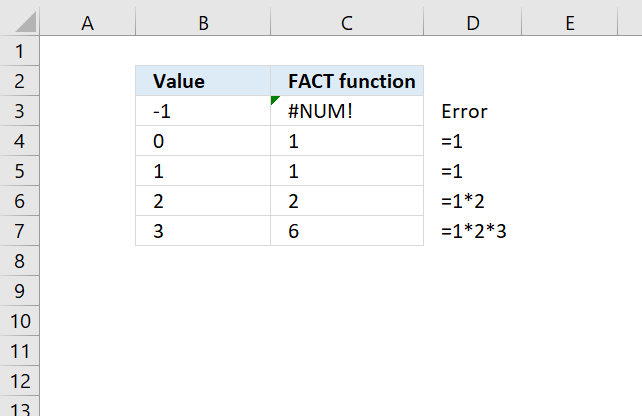Author: Oscar Cronquist Article last updated on April 14, 2018The FACT function returns the factorial of a number.

Formula in cell C3:

=FACT(B3)

Example, 3! = 3*2*1 = 6

FACT(number)

### Arguments

 number Required. The number equal to or larger than 0 (zero) for which you want to calculate the factorial.

If number is not an integer, it is truncated.

Returns an error if number argument is a text value.

The FACT function is useful if you want to calculate the number of permutations of a given set of elements.

The following set has three elements: [A, B, C].

3! = 3*2*1 = 6. You can rearrange [A, B, C] in six different permutations.

1, [A, B, C]
2, [A, C, B]
3, [B, A, C]
4, [B, C, A]
5, [C, A, B]
6, [C, B, A]